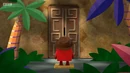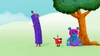WITW?No one may edit!This page is currently protected. Reason(s): Doz successfully became a BCrat!User appreciation! "I mean... hug a teddy bear?" — One This user wants to thank ThatGuy30722 with a big hug. Reason(s): giving me thread moderator This page was last edited on January 6, 2022 at 1:46 AM.

Template:FardUser appreciation! "/h/-/ʌ/-/ɡ/, HUG!" — H, U, and G This user wants to thank Dozenalism with a big hug. Reason(s): making anti-dym cid social club and giving me content moderator This page was last edited on January 6, 2022 at 1:46 AM.Cured from dymness! "No more dymness. I'm cured!" — Nine This user likes the fact that they no longer are a dym cid.This user thinks that Numberblocks toys should not be abused.The following text is to mock the immature behaviour of dym cids and is not meant to be taken seriously.

Well it looks like the dym cids are way too dym that this user decided that one DymCidMode template isn't enough to mock them.DymmestCidMode = on; And a third layer, for the dymmest cids.bfbffb driday fight hunkin is neew numberlock epis de we cn al dive xmao gous we no can ruv amogn su white teel rhs , look: seson9 will have 34 double atist, 33 a chirlider, 83 big lips, 95 quin eluzebalt, and 120 will no be step, an d 150 will be is do the setp DymmestCidMode = off;

DymmerCidMode = off;

DymCidMode = off;This user wants people to stop stealing projects around Scratch.DymCidMode = on; The following text is to mock the immature behaviour of dym cids and is not meant to be taken seriously. mom why did i get strike i want unaouthorized striek\!!!111!!!! DymCidMode = off;The super special secret Step Squad is here! Step one: Eat Pringles! That'll get you started. Step two: Show off a band! And now we're on our way. Step three: Play Among Us! Don't be downhearted. Step four: Smile :)! We're gonna save the day! Step five: We go into overdrive as we Support the LBWH, and vanish away.

Also,This user is a hater of dym cids.

the

 1 × 1 = 1 2 × 1 = 2 3 × 1 = 3 4 × 1 = 4 5 × 1 = 5 6 × 1 = 6 7 × 1 = 7 8 × 1 = 8 9 × 1 = 9 10 × 1 = 10

 1 × 2 = 2 2 × 2 = 4 3 × 2 = 6 4 × 2 = 8 5 × 2 = 10 6 × 2 = 12 7 × 2 = 14 8 × 2 = 16 9 × 2 = 18 10 × 2 = 20
 1 × 3 = 3 2 × 3 = 6 3 × 3 = 9 4 × 3 = 12 5 × 3 = 15 6 × 3 = 18 7 × 3 = 21 8 × 3 = 24 9 × 3 = 27 10 × 3 = 30

 1 × 4 = 4 2 × 4 = 8 3 × 4 = 12 4 × 4 = 16 5 × 4 = 20 6 × 4 = 24 7 × 4 = 28 8 × 4 = 32 9 × 4 = 36 10 × 4 = 40
 1 × 5 = 5 2 × 5 = 10 3 × 5 = 15 4 × 5 = 20 5 × 5 = 25 6 × 5 = 30 7 × 5 = 35 8 × 5 = 40 9 × 5 = 45 10 × 5 = 50
 1 × 6 = 6 2 × 6 = 12 3 × 6 = 18 4 × 6 = 24 5 × 6 = 30 6 × 6 = 36 7 × 6 = 42 8 × 6 = 48 9 × 6 = 54 10 × 6 = 60
 1 × 7 = 7 2 × 7 = 14 3 × 7 = 21 4 × 7 = 28 5 × 7 = 35 6 × 7 = 42 7 × 7 = 49 8 × 7 = 56 9 × 7 = 63 10 × 7 = 70
 1 × 8 = 8 2 × 8 = 16 3 × 8 = 24 4 × 8 = 32 5 × 8 = 40 6 × 8 = 48 7 × 8 = 56 8 × 8 = 64 9 × 8 = 72 10 × 8 = 80
 1 × 9 = 9 2 × 9 = 18 3 × 9 = 27 4 × 9 = 36 5 × 9 = 45 6 × 9 = 54 7 × 9 = 63 8 × 9 = 72 9 × 9 = 81 10 × 9 = 90
 1 × 10 = 10 2 × 10 = 20 3 × 10 = 30 4 × 10 = 40 5 × 10 = 50 6 × 10 = 60 7 × 10 = 70 8 × 10 = 80 9 × 10 = 90 10 × 10 = 100